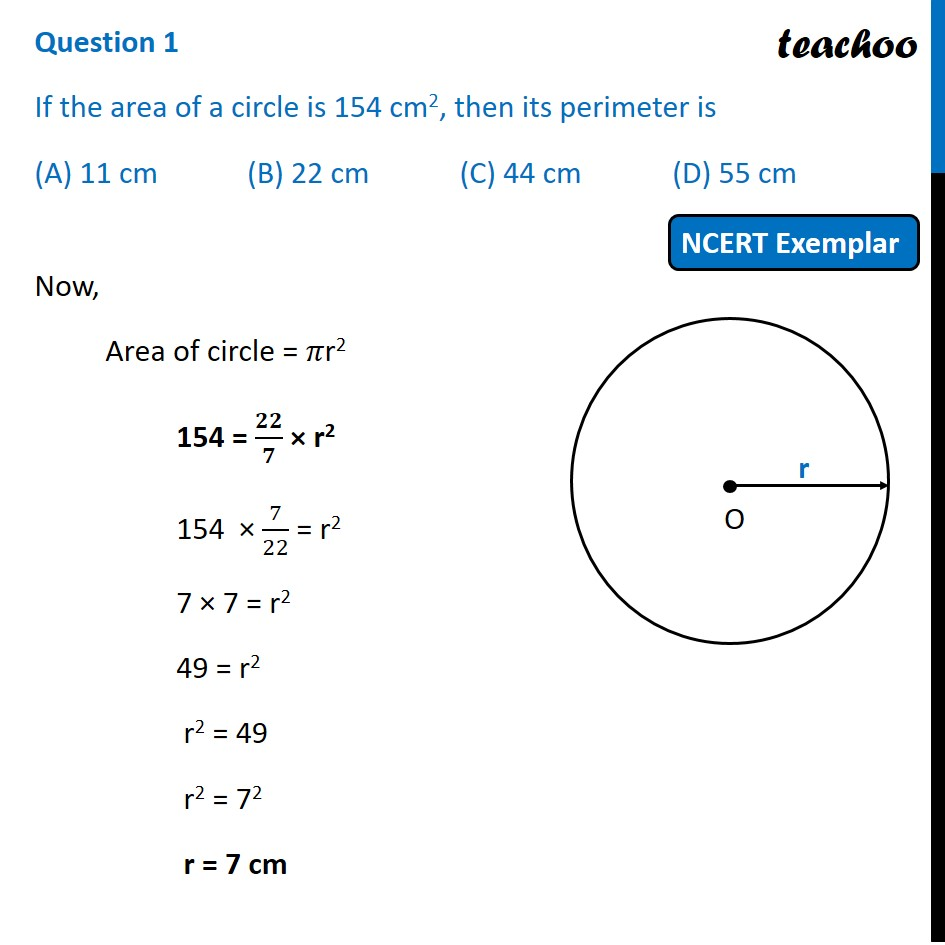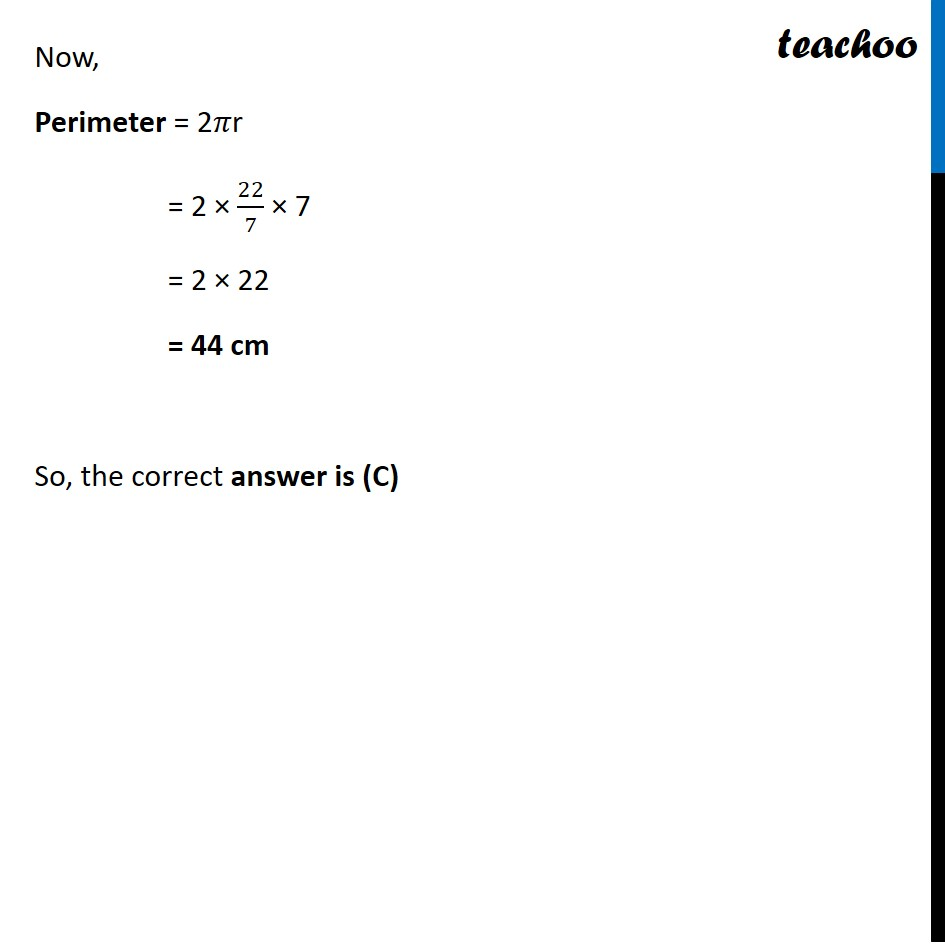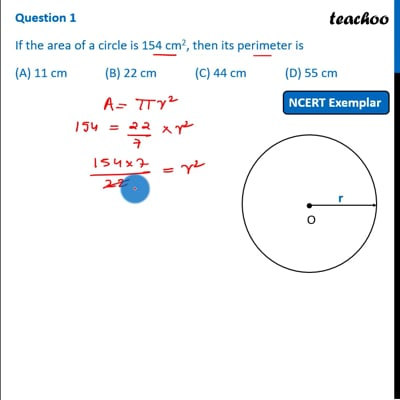NCERT Exemplar - MCQ

Chapter 12 Class 10 Areas related to Circles
Serial order wise

## (A) 11 cm   (B) 22 cm   (C) 44 cm   (D) 55 cmThis video is only available for Teachoo black users

Maths Crash Course - Live lectures + all videos + Real time Doubt solving!

### Transcript

Question 1 If the area of a circle is 154 cm2, then its perimeter is (A) 11 cm (B) 22 cm (C) 44 cm (D) 55 cm Now, Area of circle = 𝜋r2 154 = 𝟐𝟐/𝟕 × r2 154 × 7/22 = r2 7 × 7 = r2 49 = r2 r2 = 49 r2 = 72 r = 7 cm Now, Perimeter = 2𝜋r = 2 × 22/7 × 7 = 2 × 22 = 44 cm So, the correct answer is (C)